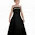## Thursday, April 7, 2011

### Energy Normalize Image in OpenCV

Hi all. Its been a while since I've written a post. In this post we discuss on how to Energy Normalize an image with given mean and variance using OpenCV.

Btw, My other programs in OpenCV will be posted here

Energy in an Image is normalized when we divide the Image pixel values with the mean value of all the pixels in the image. This is energy normalization

NOTE:
Normalizing the entire image by the mean will not give proper results (all the values from 0-255 will become values <2 or <3 (or very low values). Operating them on a block the block basis is the KEY to do it.

Other form of normalization of image you may be looking for might be Mean Normalization. There is a Mean Normalization built-in method to do it in OpenCV.

Algorithm:

image=image/mean(image);

For Block By Block processing use cvSetImageROI in Opencv (blkproc in matlab)

The code is as follows. The following returns an IplImage with energy normalized:

1.Hello Sir,

I did not understand this statement

cvConvertScale(image_32F, image_32F, (1/mean.val), 0);

what is the use and meaning of it

Thanks

2.It scales the Image/Matrix by (1/mean.val).